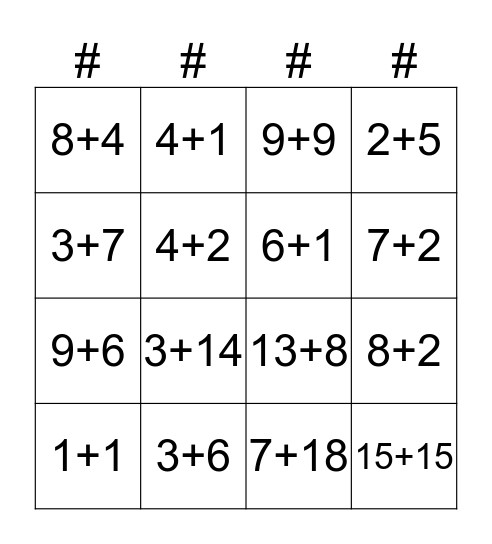This bingo card has 51 words: 1+0, 1+1, 2+1, 3+0, 3+1, 2+2, 4+1, 3+2, 4+2, 1+5, 6+1, 2+5, 4+3, 3+5, 6+2, 1+7, 7+2, 3+6, 5+4, 3+7, 8+2, 5+7, 8+4, 9+6, 10+5, 13+2, 3+14, 4+13, 10+7, 9+9, 7+11, 15+4, 6+13, 7+6, 5+8, 7+7, 9+5, 12+8, 5+15, 15+10, 7+18, 15+15, 10+20, 20+15, 5+30, 10+11, 13+8, 11+11, 16+6, 7+9 and 8+8.

## Play Online